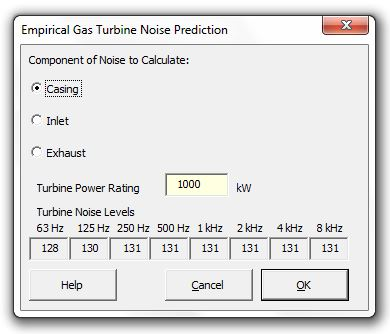### Strutt Help

Empirical Gas Turbine Noise Prediction    1/1

Strutt|Input Data|Empirical|Gas Turbine inserts sound power levels into the active row of the worksheet.The calculation of gas turbine total sound power level is split into three components:

Casing sound power is calculated by the equation:

L_W = 120 + 5log_10(MW), (dB re 10-12 W)

Inlet sound power is calculated by the equation:

L_W = 127 + 15log_10(MW), (dB re 10-12 W)

Exhaust sound power is calculated by the equation:

L_W = 133 + 10log_10(MW), (dB re 10-12 W)

The octave band sound power levels are then calculated by subtracting the corrections listed in Table 11.17 Bies and Hansen.

References:

• Bies, D., Hansen, C., Engineering Noise Control p 547